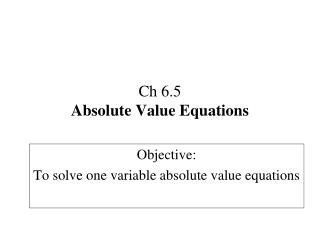Download PresentationCh 6.5 Absolute Value Equations

# Ch 6.5 Absolute Value Equations - PowerPoint PPT Presentation

Ch 6.5 Absolute Value Equations. Objective: To solve one variable absolute value equations. Definition. Absolute-Value: The distance from the origin (0). Absolute-Value Equation: An equation of the form |ax + b | = c. Rules. 1. Isolate the absolute value expressionI am the owner, or an agent authorized to act on behalf of the owner, of the copyrighted work described.
Download Presentation## Ch 6.5 Absolute Value Equations

An Image/Link below is provided (as is) to download presentation

Download Policy: Content on the Website is provided to you AS IS for your information and personal use and may not be sold / licensed / shared on other websites without getting consent from its author.While downloading, if for some reason you are not able to download a presentation, the publisher may have deleted the file from their server.

- - - - - - - - - - - - - - - - - - - - - - - - - - E N D - - - - - - - - - - - - - - - - - - - - - - - - - -
Presentation Transcript
1. Ch 6.5Absolute Value Equations Objective: To solve one variable absolute value equations

2. Definition Absolute-Value: The distance from the origin (0) Absolute-Value Equation: An equation of the form |ax + b| = c Rules 1. Isolate the absolute value expression 2. Replace the absolute value symbol | | with ± ( ) 3. Separate into two equations or inequalities a) one with the + ( ) b) one with the – ( ) 4. Solve for BOTH resulting in two answers.

3. Example 1 Example 2 = Solve = − Solve ± (x) = 5 Positive ≠ negative NOT possible! + (x) = 5 - (x) = 5 -1 -1 x = -5 No solution x = 5

4. Example 3 Solve − = ± (x - 3) = 15 + (x - 3) = 15 - (x - 3) = 15 -1 -1 - = - = - + + + + = = -

5. Example 4 Solve - = - = ± (x - 4) = 6 + (x - 4) = 6 - (x - 4) = 6 -1 -1 - = - = - = = -

6. Example 5 Solve - + = - - - = ± (2x - 3) = 25 - (2x - 3) = 25 + (2x - 3) = 25 -1 -1 - = - = - + + + + = = − = − =

7. Classwork 2) Solve |x| = −9 1) Solve |n| = 6

8. 3|x| = 9 4) Solve |n| - 4 = 1 3) Solve

9. |-8 + x| = 15 6) Solve |p + 7| = 11 5) Solve

10. 6|n - 7| = 42 8) Solve -2|m + 8| = -36 7) Solve